14 Fluid Mechanics

# 14.1 Fluids, Density, and Pressure

### Learning Objectives

By the end of this section, you will be able to:

• State the different phases of matter
• Describe the characteristics of the phases of matter at the molecular or atomic level
• Distinguish between compressible and incompressible materials
• Define density and its related SI units
• Compare and contrast the densities of various substances
• Define pressure and its related SI units
• Explain the relationship between pressure and force
• Calculate force given pressure and area

Matter most commonly exists as a solid, liquid, or gas; these states are known as the three common phases of matter. We will look at each of these phases in detail in this section.

### Characteristics of Solids

Solids are rigid and have specific shapes and definite volumes. The atoms or molecules in a solid are in close proximity to each other, and there is a significant force between these molecules. Solids will take a form determined by the nature of these forces between the molecules. Although true solids are not incompressible, it nevertheless requires a large force to change the shape of a solid. In some cases, the force between molecules can cause the molecules to organize into a lattice as shown in Figure. The structure of this three-dimensional lattice is represented as molecules connected by rigid bonds (modeled as stiff springs), which allow limited freedom for movement. Even a large force produces only small displacements in the atoms or molecules of the lattice, and the solid maintains its shape. Solids also resist shearing forces. (Shearing forces are forces applied tangentially to a surface, as described in Static Equilibrium and Elasticity.)

### Characteristics of Fluids

Liquids and gases are considered to be fluids because they yield to shearing forces, whereas solids resist them. Like solids, the molecules in a liquid are bonded to neighboring molecules, but possess many fewer of these bonds. The molecules in a liquid are not locked in place and can move with respect to each other. The distance between molecules is similar to the distances in a solid, and so liquids have definite volumes, but the shape of a liquid changes, depending on the shape of its container. Gases are not bonded to neighboring atoms and can have large separations between molecules. Gases have neither specific shapes nor definite volumes, since their molecules move to fill the container in which they are held (Figure).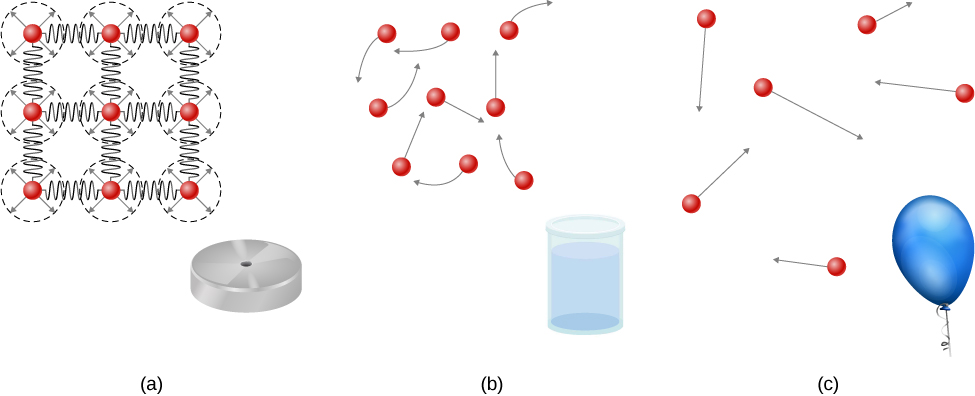Figure 14.2 (a) Atoms in a solid are always in close contact with neighboring atoms, held in place by forces represented here by springs. (b) Atoms in a liquid are also in close contact but can slide over one another. Forces between the atoms strongly resist attempts to compress the atoms. (c) Atoms in a gas move about freely and are separated by large distances. A gas must be held in a closed container to prevent it from expanding freely and escaping.

Liquids deform easily when stressed and do not spring back to their original shape once a force is removed. This occurs because the atoms or molecules in a liquid are free to slide about and change neighbors. That is, liquids flow (so they are a type of fluid), with the molecules held together by mutual attraction. When a liquid is placed in a container with no lid, it remains in the container. Because the atoms are closely packed, liquids, like solids, resist compression; an extremely large force is necessary to change the volume of a liquid.

In contrast, atoms in gases are separated by large distances, and the forces between atoms in a gas are therefore very weak, except when the atoms collide with one another. This makes gases relatively easy to compress and allows them to flow (which makes them fluids). When placed in an open container, gases, unlike liquids, will escape.

In this chapter, we generally refer to both gases and liquids simply as fluids, making a distinction between them only when they behave differently. There exists one other phase of matter, plasma, which exists at very high temperatures. At high temperatures, molecules may disassociate into atoms, and atoms disassociate into electrons (with negative charges) and protons (with positive charges), forming a plasma. Plasma will not be discussed in depth in this chapter because plasma has very different properties from the three other common phases of matter, discussed in this chapter, due to the strong electrical forces between the charges.

### Density

Suppose a block of brass and a block of wood have exactly the same mass. If both blocks are dropped in a tank of water, why does the wood float and the brass sink (Figure)? This occurs because the brass has a greater density than water, whereas the wood has a lower density than water.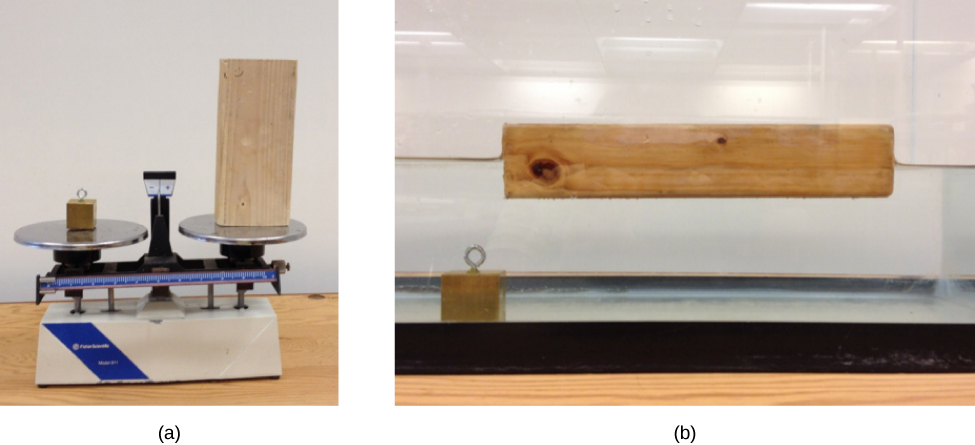Figure 14.3 (a) A block of brass and a block of wood both have the same weight and mass, but the block of wood has a much greater volume. (b) When placed in a fish tank filled with water, the cube of brass sinks and the block of wood floats. (The block of wood is the same in both pictures; it was turned on its side to fit on the scale.)

Density is an important characteristic of substances. It is crucial, for example, in determining whether an object sinks or floats in a fluid.

#### Density

The average density of a substance or object is defined as its mass per unit volume,

$\rho =\frac{m}{V}$

where the Greek letter $\rho$ (rho) is the symbol for density, m is the mass, and V is the volume.

The SI unit of density is ${\text{kg/m}}^{\text{3}}$. Figure lists some representative values. The cgs unit of density is the gram per cubic centimeter, ${\text{g/cm}}^{3}$, where

$1\,\text{g}\text{/}{\text{cm}}^{3}=1000\,\text{kg}\text{/}{\text{m}}^{3}.$

The metric system was originally devised so that water would have a density of $1\,{\text{g/cm}}^{3}$, equivalent to ${10}^{3\,}\,{\text{kg/m}}^{3}$. Thus, the basic mass unit, the kilogram, was first devised to be the mass of 1000 mL of water, which has a volume of $1000\,{\text{cm}}^{3}$.

Densities of Some Common Substances
Solids

($0.0^\circ\text{C}$)

Liquids

($0.0^\circ\text{C}$)

Gases

($0.0^\circ\text{C},$
101.3 kPa)

Substance $\rho ({\text{kg/m}}^{3})$ Substance $\rho ({\text{kg/m}}^{3})$ Substance $\rho ({\text{kg/m}}^{3})$
Aluminum $2.70\times {10}^{3}$ Benzene $8.79\times {10}^{2}$ Air $1.29\times {10}^{0}$
Bone $1.90\times {10}^{3}$ Blood $1.05\times {10}^{3}$ Carbon dioxide $1.98\times {10}^{0}$
Brass $8.44\times {10}^{3}$ Ethyl alcohol $8.06\times {10}^{2}$ Carbon monoxide $1.25\times {10}^{0}$
Concrete $2.40\times {10}^{3}$ Gasoline $6.80\times {10}^{2}$ Helium $1.80\times {10}^{-1}$
Copper $8.92\times {10}^{3}$ Glycerin $1.26\times {10}^{3}$ Hydrogen $9.00\times {10}^{-2}$
Cork $2.40\times {10}^{2}$ Mercury $1.36\times {10}^{4}$ Methane $7.20\times {10}^{-2}$
Earth’s crust $3.30\times {10}^{3}$ Olive oil $9.20\times {10}^{2}$ Nitrogen $1.25\times {10}^{0}$
Glass $2.60\times {10}^{3}$ Nitrous oxide $1.98\times {10}^{0}$
Gold $1.93\times {10}^{4}$ Oxygen $1.43\times {10}^{0}$
Granite $2.70\times {10}^{3}$
Iron $7.86\times {10}^{3}$
Lead $1.13\times {10}^{4}$
Oak $7.10\times {10}^{2}$
Pine $3.73\times {10}^{2}$
Platinum $2.14\times {10}^{4}$
Polystyrene $1.00\times {10}^{2}$
Tungsten $1.93\times {10}^{4}$
Uranium $1.87\times {10}^{3}$

As you can see by examining Figure, the density of an object may help identify its composition. The density of gold, for example, is about 2.5 times the density of iron, which is about 2.5 times the density of aluminum. Density also reveals something about the phase of the matter and its substructure. Notice that the densities of liquids and solids are roughly comparable, consistent with the fact that their atoms are in close contact. The densities of gases are much less than those of liquids and solids, because the atoms in gases are separated by large amounts of empty space. The gases are displayed for a standard temperature of $0.0^\circ\text{C}$ and a standard pressure of 101.3 kPa, and there is a strong dependence of the densities on temperature and pressure. The densities of the solids and liquids displayed are given for the standard temperature of $0.0^\circ\text{C}$ and the densities of solids and liquids depend on the temperature. The density of solids and liquids normally increase with decreasing temperature.

Figure shows the density of water in various phases and temperature. The density of water increases with decreasing temperature, reaching a maximum at $4.0^\circ\text{C,}$ and then decreases as the temperature falls below $4.0^\circ\text{C}$. This behavior of the density of water explains why ice forms at the top of a body of water.

Densities of Water
Substance $\rho ({\text{kg/m}}^{3})$
Ice $(0{}^\circ \text{C})$ $9.17\times {10}^{2}$
Water $(0^\circ\text{C})$ $9.998\times {10}^{2}$
Water $(4^\circ\text{C})$ $1.000\times {10}^{3}$
Water $(20^\circ\text{C})$ $9.982\times {10}^{2}$
Water $(100^\circ\text{C})$ $9.584\times {10}^{2}$
Steam $(100^\circ\text{C,}\,101.3\,\text{kPa})$ $1.670\times {10}^{2}$
Sea water $(0^\circ\text{C})$ $1.030\times {10}^{3}$

The density of a substance is not necessarily constant throughout the volume of a substance. If the density is constant throughout a substance, the substance is said to be a homogeneous substance. A solid iron bar is an example of a homogeneous substance. The density is constant throughout, and the density of any sample of the substance is the same as its average density. If the density of a substance were not constant, the substance is said to be a heterogeneous substance. A chunk of Swiss cheese is an example of a heterogeneous material containing both the solid cheese and gas-filled voids. The density at a specific location within a heterogeneous material is called local density, and is given as a function of location, $\rho =\rho (x,y,z)$ (Figure).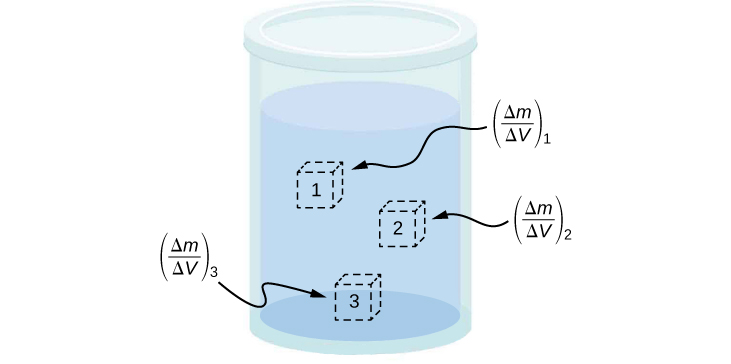Figure 14.4 Density may vary throughout a heterogeneous mixture. Local density at a point is obtained from dividing mass by volume in a small volume around a given point.

Local density can be obtained by a limiting process, based on the average density in a small volume around the point in question, taking the limit where the size of the volume approaches zero,

$\rho =\underset{\Delta V\to 0}{\text{lim}}\frac{\Delta m}{\Delta V}$

where $\rho$ is the density, m is the mass, and V is the volume.

Since gases are free to expand and contract, the densities of the gases vary considerably with temperature, whereas the densities of liquids vary little with temperature. Therefore, the densities of liquids are often treated as constant, with the density equal to the average density.

Density is a dimensional property; therefore, when comparing the densities of two substances, the units must be taken into consideration. For this reason, a more convenient, dimensionless quantity called the specific gravity is often used to compare densities. Specific gravity is defined as the ratio of the density of the material to the density of water at $4.0\,{}^\circ \text{C}$ and one atmosphere of pressure, which is $1000\,{\text{kg/m}}^{3}$:

$\text{Specific gravity}=\frac{\text{Density of material}}{\text{Density of water}}.$

The comparison uses water because the density of water is $1\,{\text{g/cm}}^{3}$, which was originally used to define the kilogram. Specific gravity, being dimensionless, provides a ready comparison among materials without having to worry about the unit of density. For instance, the density of aluminum is 2.7 in ${\text{g/cm}}^{3}$ (2700 in ${\text{kg/m}}^{3}$), but its specific gravity is 2.7, regardless of the unit of density. Specific gravity is a particularly useful quantity with regard to buoyancy, which we will discuss later in this chapter.

### Pressure

You have no doubt heard the word ‘pressure’ used in relation to blood (high or low blood pressure) and in relation to weather (high- and low-pressure weather systems). These are only two of many examples of pressure in fluids. (Recall that we introduced the idea of pressure in Static Equilibrium and Elasticity, in the context of bulk stress and strain.)

#### Pressure

Pressure (p) is defined as the normal force F per unit area A over which the force is applied, or $p=\frac{F}{A}.$

To define the pressure at a specific point, the pressure is defined as the force dF exerted by a fluid over an infinitesimal element of area dA containing the point, resulting in $p=\frac{dF}{dA}$.

A given force can have a significantly different effect, depending on the area over which the force is exerted. For instance, a force applied to an area of $1\,{\text{mm}}^{2}$ has a pressure that is 100 times as great as the same force applied to an area of $1\,{\text{cm}}^{2}.$ That is why a sharp needle is able to poke through skin when a small force is exerted, but applying the same force with a finger does not puncture the skin (Figure).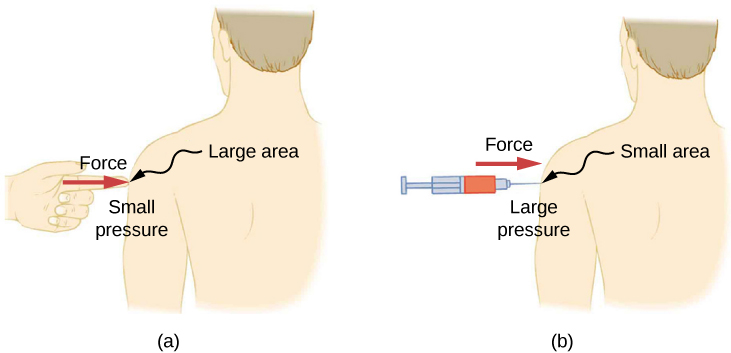Figure 14.5 (a) A person being poked with a finger might be irritated, but the force has little lasting effect. (b) In contrast, the same force applied to an area the size of the sharp end of a needle is enough to break the skin.

Note that although force is a vector, pressure is a scalar. Pressure is a scalar quantity because it is defined to be proportional to the magnitude of the force acting perpendicular to the surface area. The SI unit for pressure is the pascal (Pa), named after the French mathematician and physicist Blaise Pascal (1623–1662), where

$1\,\text{Pa}\,=1\,{\text{N/m}}^{2}.$

Several other units are used for pressure, which we discuss later in the chapter.

#### Variation of pressure with depth in a fluid of constant density

Pressure is defined for all states of matter, but it is particularly important when discussing fluids. An important characteristic of ﬂuids is that there is no significant resistance to the component of a force applied parallel to the surface of a fluid. The molecules of the ﬂuid simply ﬂow to accommodate the horizontal force. A force applied perpendicular to the surface compresses or expands the fluid. If you try to compress a fluid, you find that a reaction force develops at each point inside the fluid in the outward direction, balancing the force applied on the molecules at the boundary.

Consider a fluid of constant density as shown in Figure. The pressure at the bottom of the container is due to the pressure of the atmosphere $({p}_{0})$ plus the pressure due to the weight of the fluid. The pressure due to the fluid is equal to the weight of the fluid divided by the area. The weight of the fluid is equal to its mass times the acceleration due to gravity.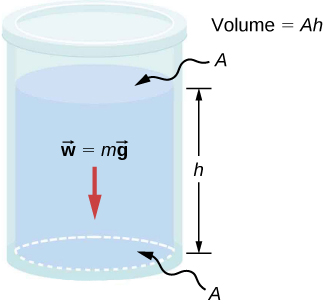Figure 14.6 The bottom of this container supports the entire weight of the fluid in it. The vertical sides cannot exert an upward force on the fluid (since it cannot withstand a shearing force), so the bottom must support it all.

Since the density is constant, the weight can be calculated using the density:

$w=mg=\rho Vg=\rho Ahg.$

The pressure at the bottom of the container is therefore equal to atmospheric pressure added to the weight of the fluid divided by the area:

$p={p}_{0}+\frac{\rho Ahg}{A}={p}_{0}+\rho hg.$

This equation is only good for pressure at a depth for a fluid of constant density.

#### Pressure at a Depth for a Fluid of Constant Density

The pressure at a depth in a fluid of constant density is equal to the pressure of the atmosphere plus the pressure due to the weight of the fluid, or

$p={p}_{0}+\rho hg,$

Where p is the pressure at a particular depth, ${p}_{0}$ is the pressure of the atmosphere, $\rho$ is the density of the fluid, g is the acceleration due to gravity, and h is the depth.Figure 14.7 The Three Gorges Dam, erected on the Yangtze River in central China in 2008, created a massive reservoir that displaced more than one million people. (credit: “Le Grand Portage”/Flickr)

### Example

#### What Force Must a Dam Withstand?

Consider the pressure and force acting on the dam retaining a reservoir of water (Figure). Suppose the dam is 500-m wide and the water is 80.0-m deep at the dam, as illustrated below. (a) What is the average pressure on the dam due to the water? (b) Calculate the force exerted against the dam.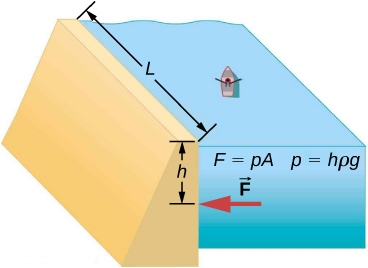The average pressure p due to the weight of the water is the pressure at the average depth h of 40.0 m, since pressure increases linearly with depth. The force exerted on the dam by the water is the average pressure times the area of contact, $F=pA.$

#### solution

1. The average pressure due to the weight of a fluid is
$p=h\rho g.$

Entering the density of water from Figure and taking h to be the average depth of 40.0 m, we obtain

$\begin{array}{cc}\hfill p& =(40.0\,\text{m})({10}^{3}\,\frac{\text{kg}}{{\text{m}}^{3}})(9.80\frac{\text{m}}{{\text{s}}^{2}})\hfill \\ & =3.92\times {10}^{5}\,\frac{\text{N}}{{\text{m}}^{2}}=392\,\text{kPa}\text{.}\hfill \end{array}$
2. We have already found the value for p. The area of the dam is
$A=80.0\,\text{m}\times 500\,\text{m}=4.00\times {10}^{4}\,{\text{m}}^{2},$

so that

$\begin{array}{}\\ \hfill F& =(3.92\times {10}^{5}\,{\text{N/m}}^{2})(4.00\times {10}^{4}\,{\text{m}}^{2})\hfill \\ & =1.57\times {10}^{10}\,\text{N}\text{.}\hfill \end{array}$

#### Significance

Although this force seems large, it is small compared with the $1.96\times {10}^{13}\text{N}$ weight of the water in the reservoir. In fact, it is only 0.0800% of the weight.

If the reservoir in Figure covered twice the area, but was kept to the same depth, would the dam need to be redesigned?

Show Solution

The pressure found in part (a) of the example is completely independent of the width and length of the lake; it depends only on its average depth at the dam. Thus, the force depends only on the water’s average depth and the dimensions of the dam, not on the horizontal extent of the reservoir. In the diagram, note that the thickness of the dam increases with depth to balance the increasing force due to the increasing pressure.

#### Pressure in a static fluid in a uniform gravitational field

A static fluid is a fluid that is not in motion. At any point within a static fluid, the pressure on all sides must be equal—otherwise, the fluid at that point would react to a net force and accelerate.

The pressure at any point in a static fluid depends only on the depth at that point. As discussed, pressure in a fluid near Earth varies with depth due to the weight of fluid above a particular level. In the above examples, we assumed density to be constant and the average density of the fluid to be a good representation of the density. This is a reasonable approximation for liquids like water, where large forces are required to compress the liquid or change the volume. In a swimming pool, for example, the density is approximately constant, and the water at the bottom is compressed very little by the weight of the water on top. Traveling up in the atmosphere is quite a different situation, however. The density of the air begins to change significantly just a short distance above Earth’s surface.

To derive a formula for the variation of pressure with depth in a tank containing a fluid of density ρ on the surface of Earth, we must start with the assumption that the density of the fluid is not constant. Fluid located at deeper levels is subjected to more force than fluid nearer to the surface due to the weight of the fluid above it. Therefore, the pressure calculated at a given depth is different than the pressure calculated using a constant density.

Imagine a thin element of fluid at a depth h, as shown in Figure. Let the element have a cross-sectional area A and height $\Delta y$. The forces acting upon the element are due to the pressures p(y) above and $p(y+\Delta y)$ below it. The weight of the element itself is also shown in the free-body diagram.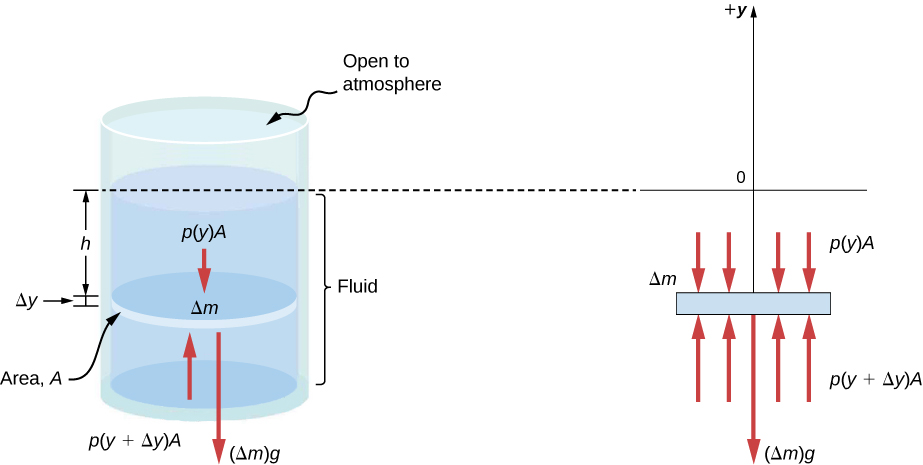Figure 14.8 Forces on a mass element inside a fluid. The weight of the element itself is shown in the free-body diagram.

Since the element of fluid between y and $y+\Delta y$ is not accelerating, the forces are balanced. Using a Cartesian y-axis oriented up, we find the following equation for the y-component:

$p(y+\Delta y)A-p(y)A-g\Delta m=0(\Delta y \gt 0).$

Note that if the element had a non-zero y-component of acceleration, the right-hand side would not be zero but would instead be the mass times the y-acceleration. The mass of the element can be written in terms of the density of the fluid and the volume of the elements:

$\Delta m=|\rho A\Delta y|=\text{−}\rho A\Delta y\text{ }(\Delta y \gt 0).$

Putting this expression for $\Delta m$ into Figure and then dividing both sides by $A\Delta y$, we find

$\frac{p(y+\Delta y)-p(y)}{\Delta y}=\text{−}\rho g.$

Taking the limit of the infinitesimally thin element $\Delta y\to 0$, we obtain the following differential equation, which gives the variation of pressure in a fluid:

$\frac{dp}{dy}=\text{−}\rho g.$

This equation tells us that the rate of change of pressure in a fluid is proportional to the density of the fluid. The solution of this equation depends upon whether the density ρ is constant or changes with depth; that is, the function ρ(y).

If the range of the depth being analyzed is not too great, we can assume the density to be constant. But if the range of depth is large enough for the density to vary appreciably, such as in the case of the atmosphere, there is significant change in density with depth. In that case, we cannot use the approximation of a constant density.

#### Pressure in a fluid with a constant density

Let’s use Figure to work out a formula for the pressure at a depth h from the surface in a tank of a liquid such as water, where the density of the liquid can be taken to be constant.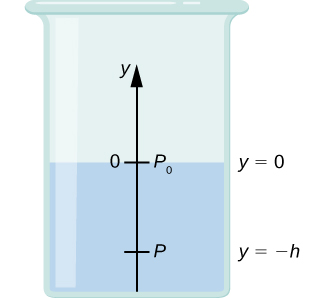We need to integrate Figure from $y=0,$ where the pressure is atmospheric pressure $({p}_{0}),$ to $y=\text{−}h,$ the y-coordinate of the depth:

$\begin{array}{ccc}\hfill {\int }_{{p}_{0}}^{p}dp& =\hfill & \text{−}{\int }_{0}^{\text{−}h}\rho gdy\hfill \\ \hfill p-{p}_{0}& =\hfill & \rho gh\hfill \\ \hfill p& =\hfill & {p}_{0}+\rho gh.\hfill \end{array}$

Hence, pressure at a depth of fluid on the surface of Earth is equal to the atmospheric pressure plus ρgh if the density of the fluid is constant over the height, as we found previously.

Note that the pressure in a fluid depends only on the depth from the surface and not on the shape of the container. Thus, in a container where a fluid can freely move in various parts, the liquid stays at the same level in every part, regardless of the shape, as shown in Figure.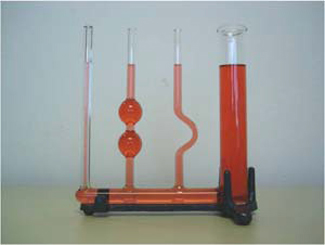Figure 14.9 If a fluid can flow freely between parts of a container, it rises to the same height in each part. In the container pictured, the pressure at the bottom of each column is the same; if it were not the same, the fluid would flow until the pressures became equal.

#### Variation of atmospheric pressure with height

The change in atmospheric pressure with height is of particular interest. Assuming the temperature of air to be constant, and that the ideal gas law of thermodynamics describes the atmosphere to a good approximation, we can find the variation of atmospheric pressure with height, when the temperature is constant. (We discuss the ideal gas law in a later chapter, but we assume you have some familiarity with it from high school and chemistry.) Let p(y) be the atmospheric pressure at height y. The density $\rho$ at y, the temperature T in the Kelvin scale (K), and the mass m of a molecule of air are related to the absolute pressure by the ideal gas law, in the form

$p=\rho \frac{{k}_{\text{B}}T}{m}\,\text{(atmosphere),}$

where ${k}_{\text{B}}$ is Boltzmann’s constant, which has a value of $1.38\times {10}^{-23}\text{J/K}$.

You may have encountered the ideal gas law in the form $pV=nRT$, where n is the number of moles and R is the gas constant. Here, the same law has been written in a different form, using the density $\rho$ instead of volume V. Therefore, if pressure p changes with height, so does the density $\rho .$ Using density from the ideal gas law, the rate of variation of pressure with height is given as

$\frac{dp}{dy}=\text{−}p(\frac{mg}{{k}_{\text{B}}T}),$

where constant quantities have been collected inside the parentheses. Replacing these constants with a single symbol $\alpha ,$ the equation looks much simpler:

$\begin{array}{ccc}\hfill \frac{dp}{dy}& =\hfill & \text{−}\alpha p\hfill \\ \hfill \frac{dp}{p}& =\hfill & \text{−}\alpha dy\hfill \\ \hfill \underset{{p}_{0}}{\overset{p(y)}{\int }}\frac{dp}{p}& =\hfill & \underset{0}{\overset{y}{\int }}\text{−}\alpha dy\hfill \\ \hfill {[\text{ln}(p)]}_{{p}_{0}}^{p(y)}& =\hfill & {[\text{−}\alpha y]}_{0}^{y}\hfill \\ \hfill \text{ln}(p)-\text{ln}({p}_{0})& =\hfill & \text{−}\alpha y\hfill \\ \hfill \text{ln}(\frac{p}{{p}_{0}})& =\hfill & \text{−}\alpha y\hfill \end{array}$

This gives the solution

$p(y)={p}_{0}\text{exp}(\text{−}\alpha y).$

Thus, atmospheric pressure drops exponentially with height, since the y-axis is pointed up from the ground and y has positive values in the atmosphere above sea level. The pressure drops by a factor of $\frac{1}{e}$ when the height is $\frac{1}{\alpha },$ which gives us a physical interpretation for $\alpha$: The constant $\frac{1}{\alpha }$ is a length scale that characterizes how pressure varies with height and is often referred to as the pressure scale height.

We can obtain an approximate value of $\alpha$ by using the mass of a nitrogen molecule as a proxy for an air molecule. At temperature $\text{27 °C,}$ or 300 K, we find

$\alpha =-\frac{mg}{{k}_{\text{B}}T}=\frac{4.8\times {10}^{-26}\,\text{kg}\,\times 9.81\,{\text{m/s}}^{2}}{1.38\times {10}^{-23}\,\text{J/K}\times \text{300 K}}=\frac{1}{8800\,\text{m}}.$

Therefore, for every 8800 meters, the air pressure drops by a factor 1/e, or approximately one-third of its value. This gives us only a rough estimate of the actual situation, since we have assumed both a constant temperature and a constant g over such great distances from Earth, neither of which is correct in reality.

#### Direction of pressure in a fluid

Fluid pressure has no direction, being a scalar quantity, whereas the forces due to pressure have well-defined directions: They are always exerted perpendicular to any surface. The reason is that fluids cannot withstand or exert shearing forces. Thus, in a static fluid enclosed in a tank, the force exerted on the walls of the tank is exerted perpendicular to the inside surface. Likewise, pressure is exerted perpendicular to the surfaces of any object within the fluid. Figure illustrates the pressure exerted by air on the walls of a tire and by water on the body of a swimmer.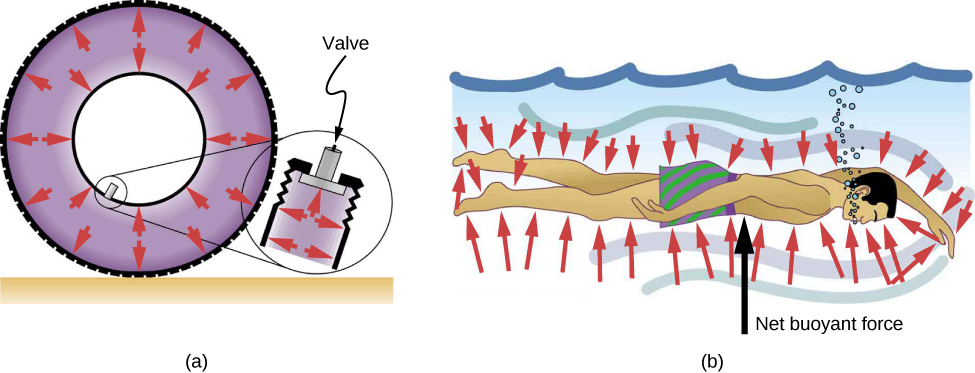Figure 14.10 (a) Pressure inside this tire exerts forces perpendicular to all surfaces it contacts. The arrows represent directions and magnitudes of the forces exerted at various points. (b) Pressure is exerted perpendicular to all sides of this swimmer, since the water would flow into the space he occupies if he were not there. The arrows represent the directions and magnitudes of the forces exerted at various points on the swimmer. Note that the forces are larger underneath, due to greater depth, giving a net upward or buoyant force. The net vertical force on the swimmer is equal to the sum of the buoyant force and the weight of the swimmer.

### Summary

• A fluid is a state of matter that yields to sideways or shearing forces. Liquids and gases are both fluids. Fluid statics is the physics of stationary fluids.
• Density is the mass per unit volume of a substance or object, defined as $\rho =m\text{/}V.$ The SI unit of density is ${\text{kg/m}}^{3}.$
• Pressure is the force per unit perpendicular area over which the force is applied, $p=F\text{/}A.$ The SI unit of pressure is the pascal: $1\,\text{Pa}=1\,{\text{N/m}}^{2}$.
• Pressure due to the weight of a liquid of constant density is given by $p=\rho \,gh$, where p is the pressure, h is the depth of the liquid, $\rho$ is the density of the liquid, and g is the acceleration due to gravity.

### Conceptual Questions

Which of the following substances are fluids at room temperature and atmospheric pressure: air, mercury, water, glass?

Show Solution

Mercury and water are liquid at room temperature and atmospheric pressure. Air is a gas at room temperature and atmospheric pressure. Glass is an amorphous solid (non-crystalline) material at room temperature and atmospheric pressure. At one time, it was thought that glass flowed, but flowed very slowly. This theory came from the observation that old glass planes were thicker at the bottom. It is now thought unlikely that this theory is accurate.

Why are gases easier to compress than liquids and solids?

Explain how the density of air varies with altitude.

Show Solution

The density of air decreases with altitude. For a column of air of a constant temperature, the density decreases exponentially with altitude. This is a fair approximation, but since the temperature does change with altitude, it is only an approximation.

The image shows a glass of ice water filled to the brim. Will the water overflow when the ice melts? Explain your answer.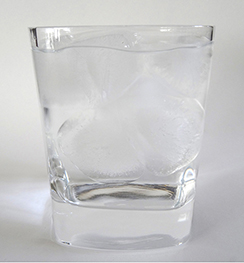How is pressure related to the sharpness of a knife and its ability to cut?

Show Solution

Pressure is force divided by area. If a knife is sharp, the force applied to the cutting surface is divided over a smaller area than the same force applied with a dull knife. This means that the pressure would be greater for the sharper knife, increasing its ability to cut.

Why is a force exerted by a static fluid on a surface always perpendicular to the surface?

Imagine that in a remote location near the North Pole, a chunk of ice floats in a lake. Next to the lake, a glacier with the same volume as the floating ice sits on land. If both chunks of ice should melt due to rising global temperatures, and the melted ice all goes into the lake, which one would cause the level of the lake to rise the most? Explain.

Show Solution

If the two chunks of ice had the same volume, they would produce the same volume of water. The glacier would cause the greatest rise in the lake, however, because part of the floating chunk of ice is already submerged in the lake, and is thus already contributing to the lake’s level.

In ballet, dancing en pointe (on the tips of the toes) is much harder on the toes than normal dancing or walking. Explain why, in terms of pressure.

Atmospheric pressure exerts a large force (equal to the weight of the atmosphere above your body—about 10 tons) on the top of your body when you are lying on the beach sunbathing. Why are you able to get up?

Show Solution

The pressure is acting all around your body, assuming you are not in a vacuum.

Why does atmospheric pressure decrease more rapidly than linearly with altitude?

The image shows how sandbags placed around a leak outside a river levee can effectively stop the flow of water under the levee. Explain how the small amount of water inside the column of sandbags is able to balance the much larger body of water behind the levee.Show Solution

Because the river level is very high, it has started to leak under the levee. Sandbags are placed around the leak, and the water held by them rises until it is the same level as the river, at which point the water there stops rising. The sandbags will absorb water until the water reaches the height of the water in the levee.

Is there a net force on a dam due to atmospheric pressure? Explain your answer.

Does atmospheric pressure add to the gas pressure in a rigid tank? In a toy balloon? When, in general, does atmospheric pressure not affect the total pressure in a fluid?

Show Solution

Atmospheric pressure does not affect the gas pressure in a rigid tank, but it does affect the pressure inside a balloon. In general, atmospheric pressure affects fluid pressure unless the fluid is enclosed in a rigid container.

You can break a strong wine bottle by pounding a cork into it with your fist, but the cork must press directly against the liquid filling the bottle—there can be no air between the cork and liquid. Explain why the bottle breaks only if there is no air between the cork and liquid.

### Problems

Gold is sold by the troy ounce (31.103 g). What is the volume of 1 troy ounce of pure gold?

Show Solution

1.610 ${\text{cm}}^{3}$

Mercury is commonly supplied in flasks containing 34.5 kg (about 76 lb.). What is the volume in liters of this much mercury?

What is the mass of a deep breath of air having a volume of 2.00 L? Discuss the effect taking such a breath has on your body’s volume and density.

Show Solution

The mass is 2.58 g. The volume of your body increases by the volume of air you inhale. The average density of your body decreases when you take a deep breath because the density of air is substantially smaller than the average density of the body.

A straightforward method of finding the density of an object is to measure its mass and then measure its volume by submerging it in a graduated cylinder. What is the density of a 240-g rock that displaces $89.0\,{\text{cm}}^{3}$ of water? (Note that the accuracy and practical applications of this technique are more limited than a variety of others that are based on Archimedes’ principle.)

Suppose you have a coffee mug with a circular cross-section and vertical sides (uniform radius). What is its inside radius if it holds 375 g of coffee when filled to a depth of 7.50 cm? Assume coffee has the same density as water.

Show Solution

3.99 cm

A rectangular gasoline tank can hold 50.0 kg of gasoline when full. What is the depth of the tank if it is 0.500-m wide by 0.900-m long? (b) Discuss whether this gas tank has a reasonable volume for a passenger car.

A trash compactor can compress its contents to 0.350 times their original volume. Neglecting the mass of air expelled, by what factor is the density of the rubbish increased?

Show Solution

2.86 times denser

A 2.50-kg steel gasoline can holds 20.0 L of gasoline when full. What is the average density of the full gas can, taking into account the volume occupied by steel as well as by gasoline?

What is the density of 18.0-karat gold that is a mixture of 18 parts gold, 5 parts silver, and 1 part copper? (These values are parts by mass, not volume.) Assume that this is a simple mixture having an average density equal to the weighted densities of its constituents.

Show Solution

$15.6\,{\text{g/cm}}^{3}$

The tip of a nail exerts tremendous pressure when hit by a hammer because it exerts a large force over a small area. What force must be exerted on a nail with a circular tip of 1.00-mm diameter to create a pressure of $3.00\times {10}^{9}\,{\text{N/m}}^{2}\text{?}$ (This high pressure is possible because the hammer striking the nail is brought to rest in such a short distance.)

A glass tube contains mercury. What would be the height of the column of mercury which would create pressure equal to 1.00 atm?

Show Solution

$0.760\,\text{m}=76.0\,\text{cm}=760\,\text{mm}$

The greatest ocean depths on Earth are found in the Marianas Trench near the Philippines. Calculate the pressure due to the ocean at the bottom of this trench, given its depth is 11.0 km and assuming the density of seawater is constant all the way down.

Verify that the SI unit of $h\rho g$ is ${\text{N/m}}^{2}$.

Show Solution

proof

What pressure is exerted on the bottom of a gas tank that is 0.500-m wide and 0.900-m long and can hold 50.0 kg of gasoline when full?

A dam is used to hold back a river. The dam has a height $H=12\,\text{m}$ and a width $W=10\,\text{m}\text{.}$ Assume that the density of the water is $\rho =1000\,{\text{kg/m}}^{3}.$ (a) Determine the net force on the dam. (b) Why does the thickness of the dam increase with depth?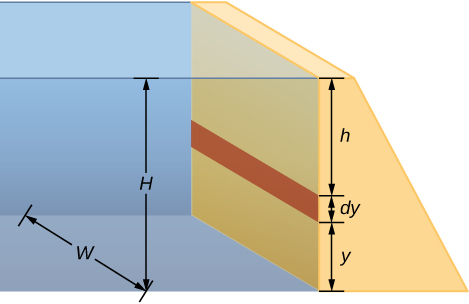Show Solution

a. Pressure at $h=7.06\times {10}^{6}\text{N}$; b. The pressure increases as the depth increases, so the dam must be built thicker toward the bottom to withstand the greater pressure.

### Glossary

density
mass per unit volume of a substance or object
fluids
liquids and gases; a fluid is a state of matter that yields to shearing forces
pressure
force per unit area exerted perpendicular to the area over which the force acts
specific gravity
ratio of the density of an object to a fluid (usually water)